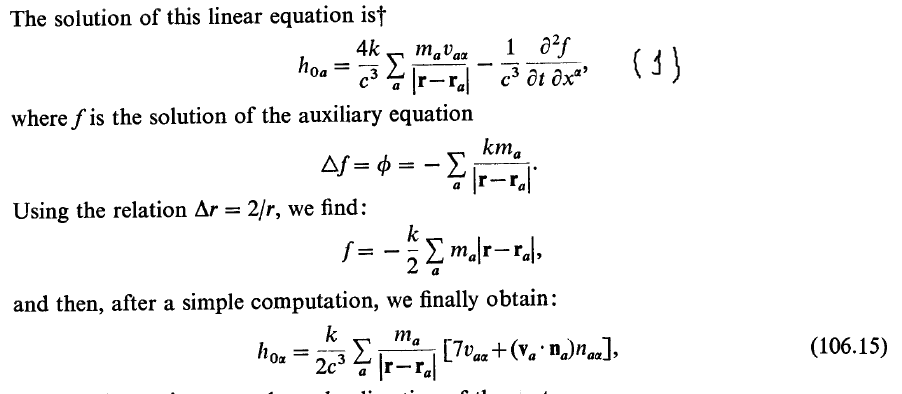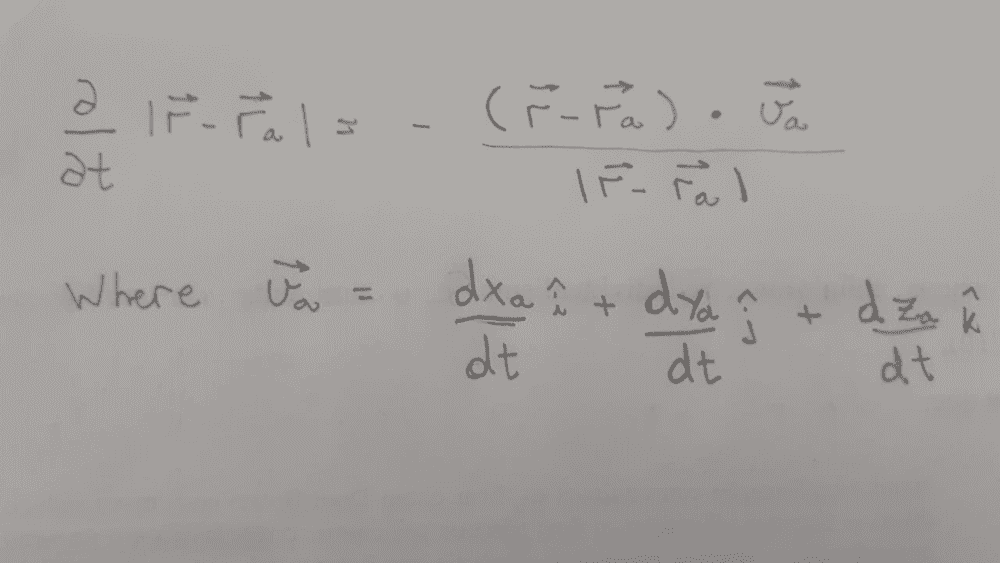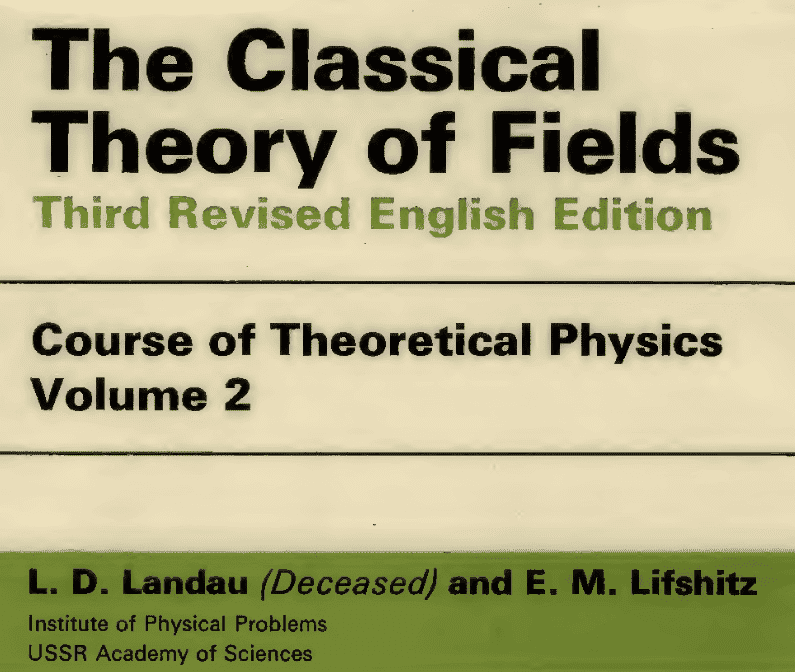# Difficulties with derivative of a vector [Landau Textbook]

• A
GrimGuy
Hi guys, I'm having trouble computing a pass 1 to 106.15. It's in the pictures.So, what a have to do is the derivative of ##f## with respect to time and coordinates. Then I need to rearrange the terms to find the equation 106.15. I am using the following conditions. ##r## vector varies in space and the vector ##r_a## varies in time.

I did that:This calculus is from. (Section 106)#### Attachments

•Delta2

The spatial dependence of ##f## is in the argument ##\mathbf{r}##, whilst the time dependence is in the source positions ##\mathbf{r}_a##\begin{align*}
\partial_{\alpha} |\mathbf{r} - \mathbf{r}_a| &= \frac{x_{\alpha} - x_{a \alpha}}{|\mathbf{r} - \mathbf{r}_a|} = n_{a \alpha}

\end{align*}Then taking the time derivative\begin{align*}
\partial_t \partial_{\alpha} |\mathbf{r} - \mathbf{r}_a| = \partial_t \left( \frac{x_{\alpha} - x_{a \alpha}}{|\mathbf{r} - \mathbf{r}_a|} \right) &= \frac{-v_{a \alpha}}{|\mathbf{r} - \mathbf{r}_a|} + (x_{\alpha} - x_{a \alpha}) \partial_t \left( \frac{1}{|\mathbf{r} - \mathbf{r}_a|} \right) \\

&= \frac{-v_{a \alpha}}{|\mathbf{r} - \mathbf{r}_a|} + \frac{-(x_{\alpha} - x_{a \alpha})}{|\mathbf{r} - \mathbf{r}_a|^2} \left( \frac{(\mathbf{r} - \mathbf{r}_a) \cdot (-\mathbf{v}_a )}{|\mathbf{r} - \mathbf{r}_a|} \right) \\

&= \frac{-v_{a \alpha}}{|\mathbf{r} - \mathbf{r}_a|} + \frac{n_{a \alpha} (\mathbf{n}_a \cdot \mathbf{v}_a)}{|\mathbf{r} - \mathbf{r}_a|}

\end{align*}Hence if ##f = -(k/2) \sum_a m_a |\mathbf{r} - \mathbf{r}_a |##, then\begin{align*}

\frac{\partial^2 f}{\partial t \partial x^a} &= \frac{k}{2} \sum_a m_a \left[ \frac{v_{a \alpha}}{|\mathbf{r} - \mathbf{r}_a|} - \frac{n_{a \alpha} (\mathbf{n}_a \cdot \mathbf{v}_a)}{|\mathbf{r} - \mathbf{r}_a|} \right]

\end{align*}and consequently\begin{align*}
h_{0 \alpha} &= \frac{8k}{2c^3} \sum_a \frac{m_a v_{a\alpha}}{|\mathbf{r} - \mathbf{r}_a|} - \frac{k}{2c^3} \sum_a \left[ \frac{m_av_{a \alpha}}{|\mathbf{r} - \mathbf{r}_a|} - \frac{m_a n_{a \alpha} (\mathbf{n}_a \cdot \mathbf{v}_a)}{|\mathbf{r} - \mathbf{r}_a|} \right] \\

&= \frac{k}{2c^3} \sum_a \frac{m_a}{|\mathbf{r} - \mathbf{r}_a|} \left[ 7v_{a \alpha} + n_{a \alpha} (\mathbf{n}_a \cdot \mathbf{v}_a ) \right]\end{align*}

Last edited by a moderator:
•GrimGuy
GrimGuy
The spatial dependence of ##f## is in the argument ##\mathbf{r}##, whilst the time dependence is in the source positions ##\mathbf{r}_a##\begin{align*}
\partial_{\alpha} |\mathbf{r} - \mathbf{r}_a| &= \frac{x_{\alpha} - x_{a \alpha}}{|\mathbf{r} - \mathbf{r}_a|} = n_{a \alpha}

\end{align*}Then taking the time derivative\begin{align*}
\partial_t \partial_{\alpha} |\mathbf{r} - \mathbf{r}_a| = \partial_t \left( \frac{x_{\alpha} - x_{a \alpha}}{|\mathbf{r} - \mathbf{r}_a|} \right) &= \frac{-v_{a \alpha}}{|\mathbf{r} - \mathbf{r}_a|} + (x_{\alpha} - x_{a \alpha}) \partial_t \left( \frac{1}{|\mathbf{r} - \mathbf{r}_a|} \right) \\

&= \frac{-v_{a \alpha}}{|\mathbf{r} - \mathbf{r}_a|} + \frac{-(x_{\alpha} - x_{a \alpha})}{|\mathbf{r} - \mathbf{r}_a|^2} \left( \frac{(\mathbf{r} - \mathbf{r}_a) \cdot (-\mathbf{v}_a )}{|\mathbf{r} - \mathbf{r}_a|} \right) \\

&= \frac{-v_{a \alpha}}{|\mathbf{r} - \mathbf{r}_a|} + \frac{n_{a \alpha} (\mathbf{n}_a \cdot \mathbf{v}_a)}{|\mathbf{r} - \mathbf{r}_a|}

\end{align*}Hence if ##f = -(k/2) \sum_a m_a |\mathbf{r} - \mathbf{r}_a |##, then\begin{align*}

\frac{\partial^2 f}{\partial t \partial x^a} &= \frac{k}{2} \sum_a m_a \left[ \frac{v_{a \alpha}}{|\mathbf{r} - \mathbf{r}_a|} - \frac{n_{a \alpha} (\mathbf{n}_a \cdot \mathbf{v}_a)}{|\mathbf{r} - \mathbf{r}_a|} \right]

\end{align*}and consequently\begin{align*}
h_{0 \alpha} &= \frac{8k}{2c^3} \sum_a \frac{m_a v_{a\alpha}}{|\mathbf{r} - \mathbf{r}_a|} - \frac{k}{2c^3} \sum_a \left[ \frac{m_av_{a \alpha}}{|\mathbf{r} - \mathbf{r}_a|} - \frac{m_a n_{a \alpha} (\mathbf{n}_a \cdot \mathbf{v}_a)}{|\mathbf{r} - \mathbf{r}_a|} \right] \\

&= \frac{k}{2c^3} \sum_a \frac{m_a}{|\mathbf{r} - \mathbf{r}_a|} \left[ 7v_{a \alpha} + n_{a \alpha} (\mathbf{n}_a \cdot \mathbf{v}_a ) \right]\end{align*}
Thx man, I'll take a time to understand and check it. Thank you a lot.

EDIT: I checked it and everything is fine and i could undestand the whole explanation, except for one little thing. What u were considering to write it '##n_{a \alpha}##'. It is the modul of the unit vector, or it is just a simplifyed notation to write ##\frac{x_{\alpha} - x_{a \alpha}}{|\mathbf{r} - \mathbf{r}_a|}## ?

Last edited:
•etotheipi
EDIT: I checked it and everything is fine and i could undestand the whole explanation, except for one little thing. What u were considering to write it '##n_{a \alpha}##'. It is the modul of the unit vector, or it is just a simplifyed notation to write ##\frac{x_{\alpha} - x_{a \alpha}}{|\mathbf{r} - \mathbf{r}_a|}## ?
Not the modulus, it's the second option: the ##\alpha## component of the vector ##\mathbf{n}_a := (\mathbf{r} - \mathbf{r}_a)/|\mathbf{r} - \mathbf{r}_a|##, as L&L define below the bit you posted. So ##n_{a \alpha} := (\mathbf{r} - \mathbf{r}_a)_{\alpha}/|\mathbf{r} - \mathbf{r}_a| = (x_{\alpha} - x_{a \alpha})/|\mathbf{r} - \mathbf{r}_a|##

I'll admit it's a slightly confusing notation on the authors' part, using ##a## to enumerate the different sources and ##\alpha## to denote vector components 😜

•GrimGuy